# 新引力宇宙度规计算星系质量和宇宙物质密度的新分析New Analysis of Calculating Galaxy’s Mass and Universe’s Material Density by New Gravity Universe Metric

DOI: 10.12677/AAS.2018.61002, PDF, HTML, XML, 下载: 1,181  浏览: 3,606

Abstract: The author gets the new equation of universe redshift and distance and uses spherical symmetry of Schwarzschild metric to get metric of irrotional black hole, which is also called new gravity cosmic metric. Together with improving Einstein gravity redshift and Newton gravity law, the author calculates the mass of galaxy again to get a new value that is only 10% of the value calculated in the literature. Through analysis, it can be learned that Newton gravity law isn’t appropriate for all galactic rotation curves and is just for limited galactic rotation curves. But this is not the final conclusion. We can’t say this analysis is useless because it has its reasonable part. New gravity cosmic metric can be used to calculate the volume of sphere that can be observed. There’s no presentation of using redshift to calculate accurate volume in Riemannian geometry. And value of cosmic horizon that was settled last century is used to figure out rational density of cosmic matter and galaxy’s mass. Mass of measurable matter is 6 times as big as that of un-measured matter. It can be learned that dark energy is accumulated by feeble gravity effect of cosmic rarefied matter. There is little dark matter in galactic halo for the mass of galaxy recal-culated by author is small. And that will bring difficulty to the direct observation. Einstein put forward the guess of unlimited universe in the 1950s. And the metric of new gravity universe is one of the solutions of his gravitational field equation, which verifies his guess. General relativi-ty dominates the whole universe.

1. 引言

2. 基础方程的导出

$r={r}_{s}\left(1-{\text{e}}^{-{z}_{U}}\right)$ ，或 ${z}_{U}=-\mathrm{ln}\left(1-r/{r}_{s}\right)$$\left(r\le {r}_{s}\right)$ (1)

$r={r}_{s}/\left(1-{\text{e}}^{-{Z}_{n}}\right)$ ，或 ${Z}_{n}=-\mathrm{ln}\left(1-{r}_{s}/r\right)$$\left(r\ge {r}_{s}\right)$ (2)

$\begin{array}{c}\text{d}{s}^{2}=-\left(1-r/{r}_{s}\right){c}^{2}\text{d}{t}^{2}+{\left(1-r/{r}_{s}\right)}^{-1}\text{d}{r}^{2}\\ +{r}^{2}\left(\text{d}{\theta }^{2}+{\mathrm{sin}}^{2}\theta \cdot \text{d}{\phi }^{2}\right)\\ =-{\text{e}}^{-{Z}_{u}}{c}^{2}\text{d}{t}^{2}+{r}_{s}{}^{2}{\text{e}}^{-{Z}_{u}}\text{d}{z}_{U}^{2}\\ +{r}_{s}{}^{2}{\left(1-{\text{e}}^{-{Z}_{u}}\right)}^{2}\left(\text{d}{\theta }^{2}+{\mathrm{sin}}^{2}\theta \text{d}{\phi }^{2}\right)\end{array}$ (3)

$\begin{array}{c}V\left({z}_{U}\right)=\underset{0}{\overset{\text{2π}}{\int }}\underset{0}{\overset{\text{π}}{\int }}\underset{0}{\overset{r}{\int }}\frac{{\text{e}}^{-{z}_{U}{}^{\prime }}{{r}^{\prime }}^{2}\mathrm{sin}\theta }{\sqrt{1-{r}^{\prime }/{r}_{s}}}\text{d}{r}^{\prime }\text{d}\theta \text{d}\phi =\left(64/105\right)\text{π}\cdot {r}_{s}^{3}\cdot {\left(1-{\text{e}}^{-{z}_{U}/2}\right)}^{3}\\ \text{\hspace{0.17em}}\text{\hspace{0.17em}}\cdot \left[1+3{\text{e}}^{-{z}_{U}/2}+6{\text{e}}^{-{z}_{U}}+\left(45/8\right){\text{e}}^{-3{z}_{U}/2}+\left(15/8\right){\text{e}}^{-2{z}_{U}}\right]\\ =\left(64/105\right)\text{π}\cdot {r}_{s}^{3}\left[1-\left(35/8\right){\text{e}}^{-1.5{z}_{U}}+\left(21/4\right){\text{e}}^{-2.5{z}_{U}}-\left(15/8\right){\text{e}}^{-3.5{z}_{U}}\right]\end{array}$ (4)

$V\left({z}_{U}\to \infty \right)=\left(64/105\right)\text{π}\cdot {r}_{s}^{3}$ ，若 ${r}_{s}$ 是经典欧氏半径，欧氏体积 ${V}_{0}=4\text{π}\cdot {r}_{s}^{3}/3$ ，比较前后体积有 $V=\left(16/35\right){V}_{0}$ 。方程(4)中不考虑宇宙物质引力效应 ${\text{e}}^{-{z}_{U}}$ 时，计算出不旋转黑洞体积或可观测宇宙实际体积，

$\begin{array}{c}{V}_{u}=\underset{0}{\overset{\text{2π}}{\int }}\underset{0}{\overset{\text{π}}{\int }}\underset{0}{\overset{r}{\int }}\frac{{{r}^{\prime }}^{2}\mathrm{sin}\theta }{\sqrt{1-{r}^{\prime }/{r}_{s}}}\text{d}{r}^{\prime }\text{d}\theta \text{d}\phi =\left(64/15\right)\text{π}\cdot {r}_{s}^{3}{\left(1-{\text{e}}^{-{z}_{U}/2}\right)}^{3}\cdot （1+9{e}^{-{z}_{U}/2}+3{e}^{-{z}_{U}}/8\right)\\ =\left(64/15\right)\text{π}{r}_{s}^{3}\left[1-\left(15/8\right){\text{e}}^{-0.5{Z}_{U}}+\left(5/4\right){\text{e}}^{-1.5{Z}_{U}}-\left(3/8\right){\text{e}}^{-2.5{Z}_{U}}\right]\end{array}$ (4a)

Einstein引力场方程的解，必含有引力，如果解不含引力则解是错误的，百年对Einstein引力场方程求解，都存在这个缺点。同时引力的数学表述不仅是Newton中心引力表述，应该还有均匀的引力表述，正是Einstein引力场方程的精准内函。在1960年Kruskal用坐标变换消除Schwarzschild度规黑洞面奇性的新度规，人们称为Kruskal度规，并出现 $r=0$ Newton引力奇性，直到现在没有天文观测验证和理论分析应用于天文观测数据，亊实上Schwarzschild度规已消除Newton引力奇性 $r=0$ ，Schwarzschild黑洞内恰是新引力宇宙度规，Schwarzschild度规黑洞面奇性不能消除，Einstein引力场方程本来自然存在的黑洞面奇性，经过大量理论分析星系观测数据验证新引力宇宙度规，才是Einstein引力场方程的正确理解。Schwarzschild 度规是中心引力度规。前者原点可以有无物质存在无关；后者原点必须在物体质量中心才有效。而且完全把宇宙红移能嵌进方程(3)度规中，引力红移方程(2)也能嵌进Schwarzschild度规中。这两个球对称度规，既可以用Einstein思路数学推导，又可以纯红移推导，结果完全相同，但文中无法分两种方法详述。

3. 星系质量新分析

$M=r{v}^{2}/G$ (5)

$\frac{2GM}{r{c}^{2}}=\frac{r}{{r}_{s}}$(6)

${r}_{Un}=\sqrt{2GM{r}_{s}/{c}^{2}}$ (7)

${r}_{Uzn}=\left(2{r}_{s}/{c}^{2}\right){v}^{2}=1.3643×{10}^{-10}{v}^{2}\text{\hspace{0.17em}}\text{kpc}$ (8)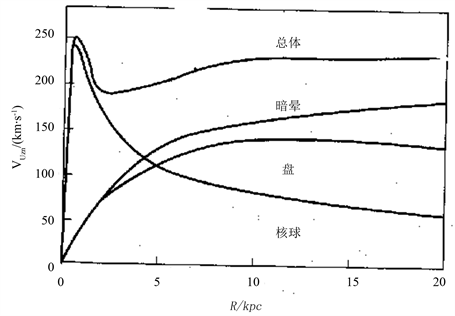Figure 1. Distribution model of mass of galaxy. The general curve used in the article is Figure 8.16 of Literature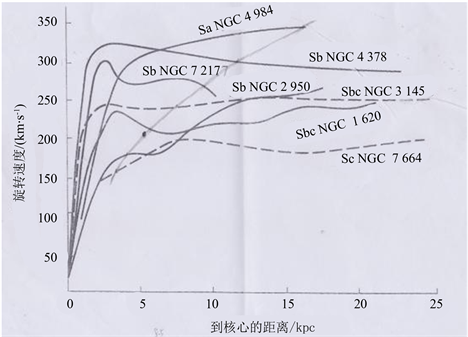Figure 2. Flat part of seven rotation curves of vortex galaxy stretches from the center to a very far place. It’s Figure 9.17 of Literature  . The curve crossing the rotation curve in the Figure is parabola of Equation (8). The dark sport is the intersection of galaxy curvesTable 1. Mass of point of galaxy and z U = z n are figured with 21 vortex galaxies and 8 point coordinates of elliptical galaxy ( r U z n , v )

In Table 1, the numbers in [*] at the top-right corner are the sequence numbers of literature. And figures of Literature  -  aren’t copied in this article.

2 × 1012Mʘ。可知计算银河系质量很混乱，没有统一的太约值。现在恆星质量计算值比较统一，百年多星系质量没有统一的太约值。原因是标准宇宙学理论无法给出计算星系质量的方程，Newton引力中心理论任意扩大应用所致。这是广义相对论推扩至全宇宙时，经过理论验证，才知上述情况。Figure 3. Black spots of Figure 6.10 and Figure 2 in Literature  are on the rotation curve (red curve) of galaxy on the right figure above. Dark spots on the parabola in Figure 2 are on the red curve of Figure (b)Table 2. There is the comparison of point coordinates ( r U z n , v ) and ( r , v ) of three vortex galaxies, which explains the existence of point coordinate ( r U z n , v )

1) 由表1知，似银河系减少很小自转速度的星系在小于 ${r}_{Uzn}$ 的质量是总质量的50％以上，那么银河系总质量约是1~1.2 × 1011Mʘ，或多一些。据此推知M31的总质量约2.6 × 1011MʘTable 3. Comparison of two equations of calculating galaxy’s mass

2) 从图2看出星系Sb NGC2950在表1中点(4.131 kpc，174 km/s)内质量约2.908 × 1010Mʘ (用方程(5)计算出)，图2该星系自转曲线上至速度250 km/s以上，离星系中心20 kpc以上，那么大于4.131 kpc以上质量约是前述质量的1~2倍，那么这星系总质量约6~9 × 1010Mʘ。可推知星系Sc NGC7664总质量约7 × 1010Mʘ

3) 表1中星系Sa NGC4984在 ${r}_{Uzn}$ 内的质量约4.5998 × 1011Mʘ，可以估计其总质量约是1012Mʘ(是前计算质量约2倍以上估算)，故表1中其余星系总质量都小于1012Mʘ。星系 $r>{r}_{Uzn}$ 的质量计算还没有统一规范，只能估算1~3倍。所以上述星系总质量上述方法估算出的。

${r}_{U{Z}_{n}}=9.430484×{10}^{-11}{v}^{2}\text{\hspace{0.17em}}\text{kpc}$ (8a)

4. 宇宙物质密度新计算和应用

4.1. 宇宙物质密度新计算

${\rho }_{0}=\frac{15{c}^{2}}{128\text{π}{r}_{s}^{2}}\approx 1.4037×{10}^{-30}\text{g}/{\text{cm}}^{\text{3}}$ (9)

${M}_{总U}=\frac{{r}_{s}{c}^{2}}{2G}=1.274×{10}^{53}\text{\hspace{0.17em}}\text{kg}$ (10)

${M}_{测U}=\left(64/105\right)\text{π}{r}_{s}^{3}{\rho }_{0}=1.820×{10}^{52}\text{\hspace{0.17em}}\text{kg}\approx 9.15×{10}^{10}$ 个星系 (11)

$P\left({z}_{U}\right)=2\text{π}\left({\text{e}}^{-1.5{z}_{U}}-2{\text{e}}^{-2.5{z}_{U}}+{\text{e}}^{-3.5{z}_{U}}\right)$ (12)

4.2. 宇宙新物质密度应用

$\begin{array}{c}{M}_{总U{Z}_{U}=2.513}={\rho }_{0}{V}_{u}=\text{π}\cdot {r}_{s}^{3}{\rho }_{0}\left(64/15\right)\left[1-\left(15/8\right){\text{e}}^{-0.5×2.513}\\ \text{\hspace{0.17em}}\text{\hspace{0.17em}}+\left(5/4\right){\text{e}}^{-1.5×2.513}-\left(3/8\right){\text{e}}^{-2.5×2.513}\right]\\ =2.109494\text{π}\cdot {r}_{s}^{3}{\rho }_{0}\end{array}$

$\begin{array}{c}{M}_{测U{Z}_{U}=2.513}={\rho }_{0}V=\text{π}{r}_{s}^{3}{\rho }_{0}\left(64/105\right)\left[1-\left(35/8\right){\text{e}}^{-1.5×2.513}\\ \text{\hspace{0.17em}}\text{\hspace{0.17em}}+\left(21/4\right){\text{e}}^{-2.5×2.513}-\left(15/8\right){\text{e}}^{-3.5×2.513}\right]\\ =0.5538277\text{π}\cdot {r}_{s}^{3}{\rho }_{0}\end{array}$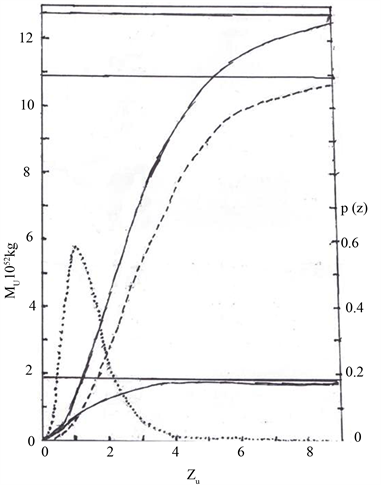Figure 4. Full curve below is ${M}_{测U}$ , dotted curve in the middle is ${M}_{总U}-{M}_{测U}$ , full curve above is ${M}_{总U}$ . The three parallel lines are asymptotes of the three mass curves and the ratio is 1.8204:10.923:12.743 = 1:6:7. Dotted line is probability curve $P\left({z}_{U}\right)$ .The vertical axis on the left is ${M}_{U}\text{\hspace{0.17em}}{10}^{52}\text{\hspace{0.17em}}\text{kg}$ and the vertical axis on the right is $P\left({z}_{U}\right)$ . The abscissa axis is redshift. The four curves are perfect, smooth and accurate theoretical curve controlled by homogeneous cosmic gravity

$\left({M}_{总U{Z}_{U}=2.513}-{M}_{测U{Z}_{U}=2.513}\right)/{M}_{测U{Z}_{U}=2.513}\approx 2.8$

$\left({V}_{u{Z}_{2}}-{V}_{u{Z}_{1}}\right){\rho }_{\Delta {Z}_{U}={\text{Z}}_{2}-{\text{Z}}_{1}}=\left({V}_{{Z}_{2}}-{V}_{{Z}_{1}}\right){\rho }_{0}$

${\rho }_{\Delta {z}_{U}={z}_{2}-{z}_{1}}=\frac{\left({V}_{{z}_{2}}-{V}_{{z}_{1}}\right){\rho }_{0}}{{V}_{u{z}_{2}}-{V}_{u{z}_{1}}}$ (13)

${\rho }_{\Delta {Z}_{U}=2.514-2.513}=1.1368×{10}^{-31}$${\rho }_{\Delta {z}_{U}=11.02-11.01}=2.3359×{10}^{-35}\text{\hspace{0.17em}}\text{g}/{\text{cm}}^{\text{3}}$ 值，这4个值清楚看出红移 $\Delta {Z}_{U}={Z}_{2}-{Z}_{1}$$V$ 球壳层处可观测物质密度随红移增加而减小。而 $V$${V}_{u}$ 的各球体物质密度 ${\rho }_{0}$ 是不变量，此情况符合宇宙观测亊实，并能精确分析可观测物质密度随红移变化规律。方程(13)的分子值是 ${V}_{u}$ 球壳层处可观测物质的数量。计算式子繁，介绍计算过程如下：方程(13)分子 ${\rho }_{0}=1.4037×{10}^{-30}\text{g}/{\text{cm}}^{\text{3}}$ ，分子小括内用方程(4)计算可观测宇宙 $V$ 球壳层体积，对 ${\rho }_{\Delta {Z}_{U}=0.009-0.008}$ 计算式如下

$\begin{array}{c}{V}_{0.009}-{V}_{0.008}=\left(64/105\right)\left[\left(35/8\right)\left({\text{e}}^{-1.5×0.008}-{\text{e}}^{-1.5×0.009}\right)-\left(21/4\right)\left({\text{e}}^{-2.5×0.008}-{\text{e}}^{-2.5×0.009}\right)\\ \text{\hspace{0.17em}}\text{\hspace{0.17em}}+\left(15/8\right)\left({\text{e}}^{-3.5×0.008}-{\text{e}}^{-3.5×0.09}\right)\right]\text{π}\cdot {r}_{s}^{3}\\ =2.8323×{10}^{-7}\text{π}\cdot {r}_{s}^{3}\end{array}$

$\begin{array}{c}{V}_{u0.009}-{V}_{u0.008}=\left(64/15\right)\left[\left(15/8\right)\left({\text{e}}^{-0.5×0.008}-{\text{e}}^{-0.5×0.009}\right)-\left(5/4\right)\left({\text{e}}^{-1.5×0.008}-{\text{e}}^{-1.5×0.009}\right)\\ \text{\hspace{0.17em}}\text{\hspace{0.17em}}+\left(3/8\right)\left({\text{e}}^{-2.5×0.008}-{\text{e}}^{-2.5×0.09}\right)\right]\text{π}\cdot {r}_{s}^{3}\\ =2.8566×{10}^{-7}\text{π}\cdot {r}_{s}^{3}\end{array}$

5. 讨论和结论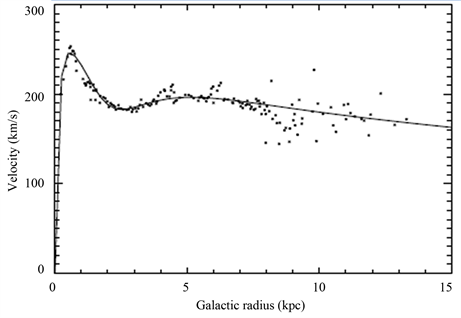Figure A1. Is copy of Galaxy’s rotation curves figure in Literature  and Literature  . After comparing, we can know that values of ${r}_{Uzn}$ of Figure A1 and Figure 1 are the same, and value of ${r}_{Uzn}$ in Figure A2 is greater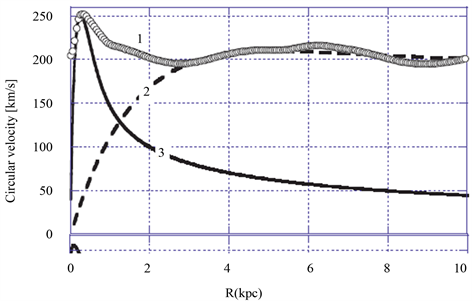Figure A2. The Figure 10 of the Literature 

Above: The rotational speeds of tracer gases in the Galaxy (Milky Way Sb spiral). Measured data from  shown in curve 1. Theoretical curve from the Carmelian equation (15) (curve 2) and from the Newtonian equation (8) (curve 3)Figure A3. The copy of Figure 3.15 in Literature  . It’s probability figure of i apparent magnitude, which has high similarity with Equation (12)

The Figure 3.15 of Literature  shows the redshift probability distributions for faint galaxies. The dashed curve shows a best fit to the measured and debiased DEEP2 redshift distribution for galaxies with i < 23, and extrapolated to i < 25 (see text).The other two curves show model predictions for galaxies with i < 25 (magenta: the Millennium Simulation; green: an evolving luminosity function; see Figure 3.14).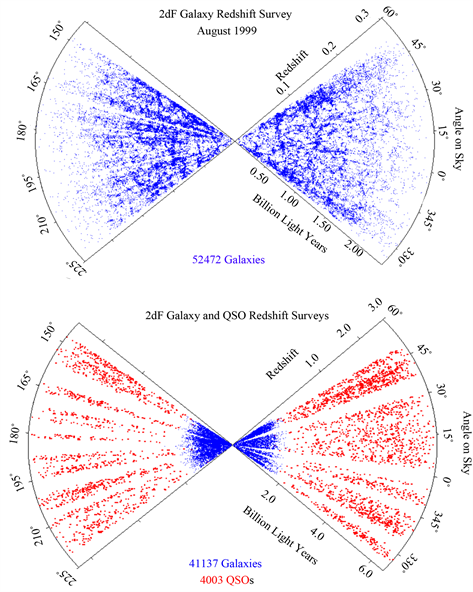Figure A4. Is the copy of Figure 2 in Literature  . It’s the two-dimen- sional figure of redshift’s distribution of galaxy and quasar, which is in accord with the distributing rule of Equation (12) and Equation (13). Quantity of point coordinate decreases as redshift increases, due to that density of observed matter decreases as redshift increases

We can see from this picture, redshift cone diagram showing the distribution of objects from the 2dF galaxy and QSO redshift surveys as of August 1999. The top panel shows the distribution of the galaxies alone, and the bottom panel shows the distributions of both galaxies and QSOs.

  何香涛. 观测宇宙学[M]. 第2版. 北京: 北京师范大学出版社, 2007.  李宗伟, 肖兴华. 天体物埋学[M]. 北京: 高等教育出版社, 2012: 370.  Pizzella, A., Corsini, E.M., Dalla Bonta1, E. and Bertola, F. (2005) On the Relation between Circular Velocity and Central Velocity Dispersion in High and Low Surface Brightness Gal-axies. The Astrophysical Journal, 631, 785-791. http://arxiv.org/abs/astro-ph/0503649v2  Dias, W.S. and Lepine, J.R.D. (2005) Direct Determination of the Spiral Pattern Rotation Speed of the Galaxy. The Astrophysical Journal, 629, 825-831. http://arxiv.org/abs/astro-ph/0503083v1 https://doi.org/10.1086/431456  Widrow, L.M. and Dubinski, J. (2005) Equilibrium Disk-Bulge-Halo Models for the Milky Way and Andromeda Galaxies. The Astrophysical Journal, 631, 838-855. http://arxiv.org/abs/astro-ph/0506177v1 https://doi.org/10.1086/432710  Hartnett, J.G. (2006) Spiral Galaxy Rotation Curves Determined from Carmelian General Relativity. International Journal of Theoretical Physics, 45, 2118-2006. http://arxiv.org/abs/astro-ph/0511756v3 https://doi.org/10.1007/s10773-006-9178-0  Blais-Ouellette, S., Amram, P., Carignan, C. and Swaters, R. (2004) Accurate Determination of the Mass Distribution in Spiral Galaxies: 3. Fabry-Perot Imaging Spectros-copy of 6 Spiral Galaxies. Astronomy & Astrophysics Journal, 420, 147-161. http://arxiv.org/pdf/astro-ph/0411001.pdf  Shalyapina, L.V., Moiseev, A.V., Yakovleva1, V.A., et al. (2004) The Phenomenon of the Galaxy NGC 6286: A Forming Polar Ring or a Superwind? Astron.Lett.J., 30, 1-13. http://arxiv.org/abs/astro-ph/0312564v1  向守平. 天体物埋学概论[M]. 第2版. 合肥: 中国科技大学出版社, 2012: 209.  陆埮, 主编. 现代天体物理(上)[M]. 北京: 北京大学出版社, 201: 6 22 135 198.  Binney, J. and Tremaine, S. Galactic Dynamics [M]. 宋国玄, 译. 上海: 上海科技出版社, 2005: 37-63.  Marr, J.H. (2015) Angular Momentum of Disc Galaxies with a Lognormal Density Distribution. MNRAS, 453, 2214- 2219. http://lanl.arxiv.org/abs/1507.04515 https://doi.org/10.1093/mnras/stv1734  Weinberg, S. Cosmology [M]. 向守平, 译. 合肥: 中国科学技术大学出版社, 2013: 26-83.  朱慈墭. 天文学教程: 下冊[M]. 第二版. 北京: 高等教育出版社, 2003.  The LSST Science Collaborations, USA LSST Science Book. The Large Synoptic Survey Telescope. http://www.lsst.org/  Colless, M. Redshift Surveys and Cosmology for this version. arXiv:astro-ph/9911326v1  Gasanalizade, A.G. On Synthesis of the Big Bang Model with Freundlich’s Redshift and Its Cosmological Consequences. http://arxiv.org/abs/astro-ph/1009.4775  黄洵. 新引力宇宙度规在星系光度和星系团的验证[J]. 天文与天体物理, 2016, 4(4): 69-80.  黄洵. 新引力宇宙度规[EB/OL]. 中国科学院科学智慧火花. http://idea.cas.cn/viewdoc.action?docid=40374, 2015- 10-21.  黄洵. 引力透镜新应用[EB/OL]. 中国科学院科学智慧火花. http://idea.cas.cn/viewdoc.action?docid=34599, 2015- 04-20.  黄洵. 经典距离与光锥距离的应用分析——中科院科学智慧火花[EB/OL]. http://idea.cas.cn/viewdoc.action?docid=38375, 2015-08-17.